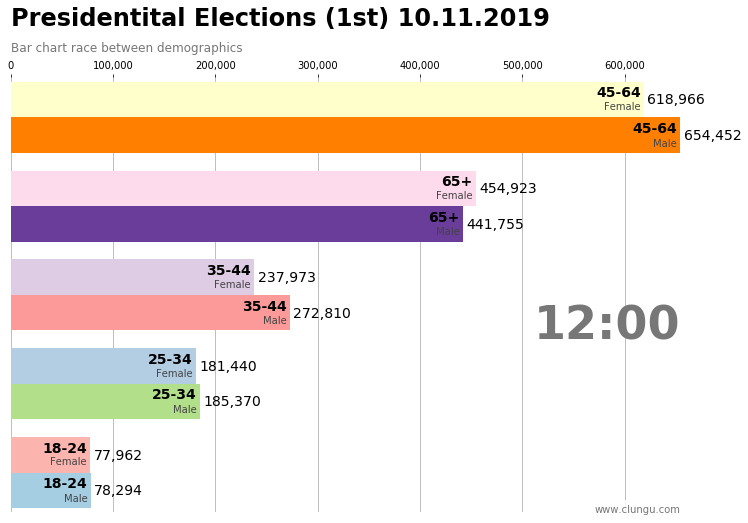# Presidential_elections_visualisations

As I’ve said last time we’re going to do some visualization exercises by using `matplotlib` animation and some custom made charts.

During the first round of the presidential election, I’ve collected the attendance data (as was described here) and I plan to use that as a source for creating two types of graphs:

• Bar chart race of some bucketed demographics
• Choropleth graphs of the attendance

Although the goal of this post is not draw hard conclusions about what these will show, some comments and interesting insight will be inlined. I’m sure though that a more careful look at these charts and graphs could yield more insights that I could offer so feel free to interpret them on your own.

# Bar chart race of demographics

## Bar charts race graphs

Bar chart races are a niche but not that new form of visualization techniques. They started being discussed more often after Matt Navarra’s tweet, which was viewed 10 million times. We then had John Burn-Murdoch’s implementation in d3.js and Pratap Vardhan’s matplotlib implementation.

I’m going to do a derivate of Pretap’s version since it doesn’t involve other dependencies and is really well suited to a data scientist’s tool box: `Python, Numpy, Pandas, Matplotlib`.

## Race of demographics

The dataset was already cleaned and aggregated beforehand. It consists of total votes for the following categories, taken throughout the day:

• 18-24, male / female
• 25-34, male / female
• 35-44, male / female
• 45-64, male / female
• 65+, male / female
Code
``````import numpy as np
import pandas as pd
import geopandas as gpd
from matplotlib import pyplot as plt
``````
``````df_by_age = pd.read_parquet("./_data/df_by_age.parquet")
df_by_age = df_by_age.reset_index()
``````
hour minute second Barbati 18-24 Barbati 25-34 Barbati 35-44 Barbati 45-64 Barbati 65+ Femei 18-24 Femei 25-34 Femei 35-44 Femei 45-64 Femei 65+
0 10 1 40 35901 93293.0 139520.0 343678.0 214137.0 35100.0 83519.0 111685.0 296501.0 197246.0
1 10 1 51 35901 93293.0 139520.0 343678.0 214137.0 35100.0 83519.0 111685.0 296501.0 197246.0
2 10 2 2 35901 93293.0 139520.0 343678.0 214137.0 35100.0 83519.0 111685.0 296501.0 197246.0
3 10 5 38 36629 94938.0 141966.0 351276.0 220240.0 35795.0 85116.0 113991.0 303835.0 203092.0
4 10 6 49 37136 96125.0 143825.0 356515.0 224211.0 36251.0 86249.0 115569.0 308857.0 207084.0

We’re going to get a single row of data at a time and compile a single frame of the animation. These will be later tied together into a continuous one.

Code
``````def get_row(i):
"""
Gets the data about a single row
"""
hour, minute, second = df_by_age.iloc[i][['hour', 'minute', 'second']].astype(int).values
_males = df_by_age.iloc[i][males].astype(int).values
_females = df_by_age.iloc[i][females].astype(int).values
return hour, minute, second, _males, _females

get_row(0)
``````
``````(10,
1,
40,
array([ 35901,  93293, 139520, 343678, 214137]),
array([ 35100,  83519, 111685, 296501, 197246]))
``````

Let’s see how a single frame will look like:

Code
``````import matplotlib.ticker as ticker
from matplotlib.cm import get_cmap
from sklearn.preprocessing import normalize
from matplotlib.colors import Normalize
fig, ax = plt.subplots(figsize=(12, 8))

title = 'Presidentital Elections (1st) 10.11.2019'
subtitle = 'Bar chart race between demographics'

demographics = set(df_by_age.columns) - set(['hour', 'minute', 'second'])
males = np.array(sorted({category for category in demographics if "Barbati" in category}))
females = np.array(sorted({category for category in demographics if "Femei" in category}))

norm = Normalize(vmin=0, vmax=len(males))
female_cmap = get_cmap('Pastel1')
male_cmap = get_cmap('Paired')
male_xticks = np.arange(len(males))-0.2
female_xticks = np.arange(len(males))+0.2

def single_frame(i, ax, title, subtitle):

hour, minute, second, _males, _females = get_row(i)

male_order = np.argsort(_males)
female_order = np.argsort(_females)

ax.clear()
ax.barh(male_xticks, _males[male_order], height=0.4, color=[to_hex(male_cmap(norm(i))) for i in male_order])
ax.barh(female_xticks, _females[female_order], height=0.4, color=[to_hex(female_cmap(norm(i))) for i in female_order])

ax.text(0, 1.12, title, transform=ax.transAxes, size=24, weight=600, ha='left')
ax.text(0, 1.06, subtitle, transform=ax.transAxes, size=12, color='#777777')

dx = max(_males.max(), _females.max()) / 200
for i, (value, name) in enumerate(zip(_males[male_order], males[male_order])):
ax.text(value-dx, i-0.2, name.replace("Barbati ", ""), size=14, weight=600, ha='right', va='bottom')
ax.text(value-dx, i-.32, "Male", size=10, color='#444444', ha='right', va='baseline')
ax.text(value+dx, i-0.2, f'{value:,.0f}',  size=14, ha='left',  va='center')

for i, (value, name) in enumerate(zip(_females[female_order], females[female_order])):
ax.text(value-dx, i+0.2, name.replace("Femei ", ""), size=14, weight=600, ha='right', va='bottom')
ax.text(value-dx, i+.09, "Female", size=10, color='#444444', ha='right', va='baseline')
ax.text(value+dx, i+0.2, f'{value:,.0f}',  size=14, ha='left',  va='center')

hour_string = f"{hour}:{minute}" if minute > 9 else f"{hour}:0{minute}"
ax.text(1, 0.4, hour_string, transform=ax.transAxes, color='#777777', size=46, ha='right', weight=800)
ax.text(1, 0, 'www.clungu.com', transform=ax.transAxes, ha='right',
color='#777777', bbox=dict(facecolor='white', alpha=0.8, edgecolor='white'))

ax.xaxis.set_major_formatter(ticker.StrMethodFormatter('{x:,.0f}'))
ax.xaxis.set_ticks_position('top')
ax.grid(which='major', axis='x', linestyle='-')

ax.set_yticks([])

ax.set_axisbelow(True)
ax.margins(0, 0.01)
plt.box(False)

single_frame(100, ax, title, subtitle)
``````And the final result is shown bellow:

Code
``````import matplotlib.animation as animation
from IPython.display import HTML, display

title = 'Presidentital Elections (1st) 10.11.2019'
subtitle = 'Bar chart race between demographics'
frames = df_by_age.shape

fig, ax = plt.subplots(figsize=(12, 8))
single_frame(0, ax, title, subtitle)
fig.tight_layout()

animator = animation.FuncAnimation(fig, single_frame, fargs=(ax, title, subtitle), frames=frames, interval=50, blit=False)
display(HTML(animator.to_html5_video()))
plt.close()
``````